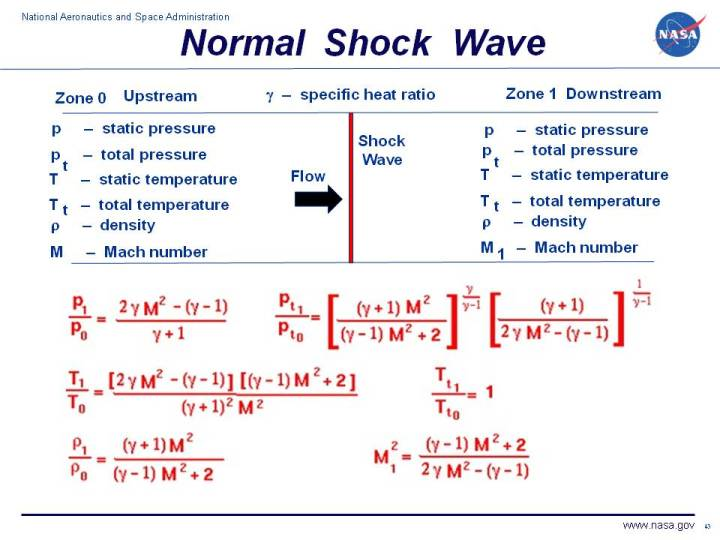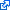+ Text Only Site
+ Non-Flash Version
+ Contact GlennAs an object moves through a gas, the gas molecules are deflected around the object. If the speed of the object is much less than the speed of sound of the gas, the density of the gas remains constant and the flow of gas can be described by conserving momentum and energy. As the speed of the object increases towards the speed of sound, we must consider compressibility effects on the gas. The density of the gas varies locally as the gas is compressed by the object. For compressible flows with little or small flow turning, the flow process is reversible and the entropy is constant. The change in flow properties are then given by the isentropic relations Isentropic means "constant entropy". But when an object moves faster than the speed of sound, and there is an abrupt decrease in the flow area, the flow process is irreversible and the entropy increases. Shock waves are generated. Shock waves are very small regions in the gas where the gas properties change by a large amount. Across a shock wave, the static pressure, temperature, and gas density increases almost instantaneously. Because a shock wave does no work, and there is no heat addition, the total enthalpy and the total temperature are constant. But because the flow is non-isentropic, the total pressure downstream of the shock is always less than the total pressure upstream of the shock. There is a loss of total pressure associated with a shock wave. The ratio of the total pressure is shown on the slide. Because total pressure changes across the shock, we can not use the usual (incompressible) form of Bernoulli's equation across the shock. The Mach number and speed of the flow also decrease across a shock wave. If the shock wave is perpendicular to the flow direction it is called a normal shock. On this slide we have listed the equations which describe the change in flow variables for flow across a normal shock. The equations presented here were derived by considering the conservation of mass, momentum, and energy. for a compressible gas while ignoring viscous effects. The equations have been further specialized for a one-dimensional flow without heat addition. A normal shock occurs in front of a supersonic object if the flow is turned by a large amount and the shock cannot remain attached to the body. The detached shock occurs for both wedges and cones. A normal shock is also present in most supersonic inlets. Across the normal shock the flow changes from supersonic to subsonic conditions. Since gas turbine engines operate under subsonic conditions, it is necessary to introduce a normal shock in the inlet compression system. Normal shocks also are generated in shock tubes. A shock tube is a high velocity wind tunnelin which the temperature jump across the normal shock is used to simulate the high heating environment of spacecraft re-entry. Across the normal shock wave the Mach number decreases to a value specified as M1: M1^2 = [(gam - 1) * M^2 + 2] / [2 * gam * M^2 - (gam - 1)] where gam is the ratio of specific heats and M is the upstream Mach number. The total temperature Tt across the shock is constant, Tt1 / Tt0 = 1 The static temperature T increases in zone 1 to become: T1 / T0 = [2 * gam * M^2 - (gam - 1)] * [(gam - 1) * M^2 + 2] / [(gam + 1)^2 * M^2] The static pressure p increases to: p1 / p0 = [2* gam * M^2 - (gam - 1)] / (gam + 1) And the density r changes by: r1 / r0 = [(gam + 1) * M^2 ] / [(gam -1 ) * M^2 + 2] The total pressure pt decreases according to: pt1 / pt0 = {[(gam + 1) * M^2 ] / [(gam - 1) *M^2 + 2]}^[gam/(gam-1)] * {(gam + 1) /[2 * gam * M^2 - (gam - 1)]}^[1/(gam - 1)] The right hand side of all these equations depend only on the free stream Mach number. So knowing the Mach number, we can determine all the conditions associated with the normal shock. The equations describing normal shocks were published in a NACA report (NACA-1135) in 1951. Here's a Java program based on the normal shock equations. Due to IT security concerns, many users are currently experiencing problems running NASA Glenn educational applets. The applets are slowly being updated, but it is a lengthy process. If you are familiar with Java Runtime Environments (JRE), you may want to try downloading the applet and running it on an Integrated Development Environment (IDE) such as Netbeans or Eclipse. The following are tutorials for running Java applets on either IDE: NetbeansEclipseThis page shows an interactive Java applet to calculate the normal shock relations. To change input values, click on the input box (black on white), backspace over the input value, type in your new value. Then hit the red COMPUTE button to send your new value to the program. You will see the output boxes (yellow on black) change value. You can use either Imperial or Metric units and you can set default conditions for the Earth or Mars. Just click on the menu button and click on your selection. For the given altitude input, the program computes the upstream standard day conditions. The ratios are computed using the Mach number and specific heat ratio inputs and the equations given in the figure. The downstream conditions are computed from the upstream conditions and the ratios. If you are an experienced user of this simulator, you can use a sleek version of the program which loads faster on your computer and does not include these instructions. You can also download your own copy of the program to run off-line by clicking on this button: There is more complete shock simulation program that is available at this web site. This program solves for the shocks generated by a single wedge or cone, including the detached normal shock condition. Another shock simulation, called ShockModeler, describes the intersection and reflection of multiple shock waves. Activities: Guided ToursCompressible Aerodynamics:Shock Waves and Expansions:Single Shock Wave Simulator:Multiple Shock Wave Simulator:Sources of Drag:Inlet:Navigation ..Beginner's Guide Home Page+ Inspector General Hotline + Equal Employment Opportunity Data Posted Pursuant to the No Fear Act + Budgets, Strategic Plans and Accountability Reports + Freedom of Information Act + The President's Management Agenda + NASA Privacy Statement, Disclaimer, and Accessibility CertificationEditor: Nancy Hall NASA Official: Nancy Hall Last Updated: May 13 2021 + Contact Glenn